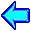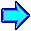#AP Computer Science Workshop

C++ Caveats

Confusing = and ==
if (x=0) {cout << "never executed" << endl;}

Arrays always begin at 0
apvector<int> a(100);
for (k=0; k<100; k++) {a[k] = k;}

Short circuited boolean evaluation
while (k < size && a[k] != value) {k++;}Mark Stehlik, Carnegie Mellon University